## Oblate Spheroid Geodesic

The Geodesic on an Oblate Spheroid can be computed analytically for a spheroid specified parametrically by(1)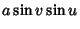(2)(3)

with, although it is much more unwieldy than for a simple Sphere. Using the first Partial Derivatives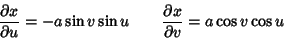(4)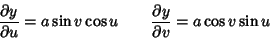(5)(6)

and second Partial Derivatives(7)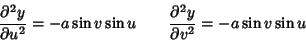(8)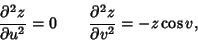(9)

gives the Geodesics functions as(10)(11)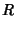(12)

Sinceandandare explicit functions ofonly, we can use the special form of the Geodesic equation.(13)

Integrating gives(14)

where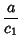(15)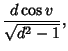(16)is an Elliptic Integral of the First Kind with Parameter, andis an Elliptic Integral of the Third Kind.

Geodesics other than Meridians of an Oblate Spheroid undulate between two parallels with latitudes equidistant from the equator. Using the Weierstraß Sigma Function and Weierstraß Zeta Function, the Geodesic on the Oblate Spheroid can be written as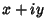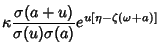(17)(18)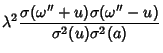(19)

(Forsyth 1960, pp. 108-109; Halphen 1886-1891).

The equation of the Geodesic can be put in the form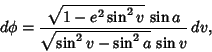(20)

whereis the smallest value ofon the curve. Furthermore, the difference in longitude between points of highest and next lowest latitude on the curve is(21)

where the Modulus of the Elliptic Function is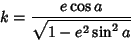(22)

(Forsyth 1960, p. 446).